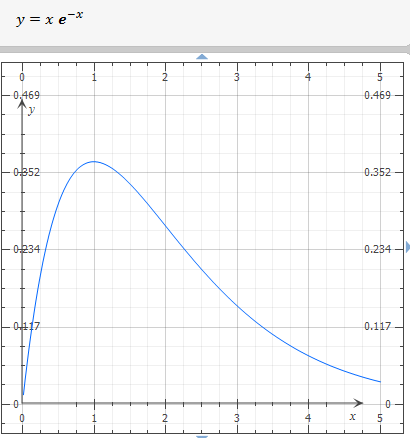# Consider the function f(x) = xe^{-x} on the interval [0, \infty). Follow these steps to graph the...

## Question:

Consider the function {eq}f(x) = xe^{-x} {/eq} on the interval {eq}[0, \infty) {/eq}. Follow these steps to graph the function.

(a) The domain of f is _____.

(b) The derivative of f is f' = _____

(c) The critical point(s) of f are _____.

(d) The function is increasing on the interval _____.

(e) The second derivative of f is f" = _____

(f) The function is concave upward on the interval _____. The function is concave downward on the interval _____.

(g) The point(s) of inflection of f are _____.

(h) The graph of f for {eq}x \in [0,5] {/eq} is _____.

## Increasing and Decreasing Intervals

A function with one independent variable has increasing intervals if its first derivative is positive, and decreasing intervals if its first derivative is negative, also, a function is concave up if its second derivative is positive and concave down if its second derivative is negative.

(a) The domain of f is:

{eq}\displaystyle x \; \in \mathbb{R} \; \text{ or } \; x \; \in \; (-\infty, \infty) {/eq}

(b) The derivative of f is:

{eq}\displaystyle \ f'(x) \; = \; {{\rm e}^{-x}}-x{{\rm e}^{-x}} \\ \displaystyle \ f'(x) \; = \; -{{\rm e}^{-x}} \left( x-1 \right) \\ {/eq}

(c) The critical point(s) of f are:

{eq}\displaystyle 0= \ f'(x) \; \; \Rightarrow \; 0= -{{\rm e}^{-x}} \left( x-1 \right) \; \Leftrightarrow \; x= 1 \\ {/eq}

(d) The function is increasing on:

First derivative test:

{eq}\begin{array} \; \text{Interval} \; & { -\infty < x < 1 } & { 1 < x < \infty } \\ \hline Test \space{} value & { x = -11 } & { x = 11 } \\ Test & { f'( -11 ) = + > 0 } & { f'( 11 ) = - < 0 } \\ Conclusion & { increasing } & { decreasing } \\ \end{array} \\ \therefore \text{ increasing's interval is} \; \ ( -\infty , 1 ) \\ \therefore \text{ decreasing's interval is} \; \ ( 1 , \infty ) \\ {/eq}

(e) The second derivative of f is:

{eq}\displaystyle \ f''(x) \; = \; -2\,{{\rm e}^{-x}}+x{{\rm e}^{-x}} \\ \displaystyle \ f''(x) \; = \; {{\rm e}^{-x}} \left( x-2 \right) \\ {/eq}

(f) Concavity behavior:

{eq}\begin{array} \; \text{Interval} \; & { -\infty < x < 2 } & { 2 < x < \infty } \\ \hline Test \space{} value & { x = -5 } & { x = 5 } \\ Test & { f''( -5 ) = - < 0 } & { f''( 5 ) = + > 0 } \\ Conclusion & { concave \; downward } & { concave \; upward } \\ \end{array} \\ \therefore \boxed { \text{ concave upward interval is} \; \ ( 2 , \infty )} \\ \therefore \boxed { \text{ concave downward interval is} \; \ ( -\infty , 2 )} \\ {/eq}

(g) Infelction point(s)

{eq}\displaystyle \ f''(x) \; = \;0 \; \Rightarrow \; {{\rm e}^{-x}} \left( x-2 \right) =0 \; \Leftrightarrow \; x=2 \\ \displaystyle (2,f(2)) \; \Rightarrow \; (2, 0.2706705664) {/eq}

(h) The graph of f for {eq}x \in [0,5] {/eq} is: